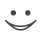# This flashcard is just one of a free flashcard set. See all flashcards!

135
Which of the following is NOT true about duration?
A.It equals the years-to-maturity for a zero coupon bond
B.It equals the weighted average of payment times for a bond, where weights are proportional to the present value of payments
C.Equals the weighted average of individual bond durations for a portfolio, where weights are proportional to the present value of bond prices
D.The prices of two bonds with the same duration change by the same percentage amount when interest rate moves up by 100 basis points